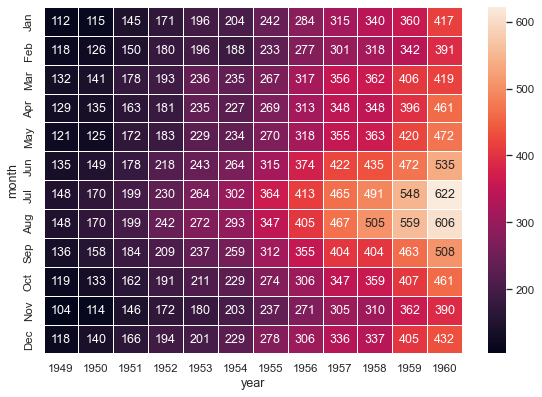# Annotated heatmaps¶seaborn components used: `set_theme()`, `load_dataset()`, `heatmap()`

```import matplotlib.pyplot as plt
import seaborn as sns
sns.set_theme()

# Load the example flights dataset and convert to long-form
flights_long = sns.load_dataset("flights")
flights = flights_long.pivot("month", "year", "passengers")

# Draw a heatmap with the numeric values in each cell
f, ax = plt.subplots(figsize=(9, 6))
sns.heatmap(flights, annot=True, fmt="d", linewidths=.5, ax=ax)
```